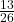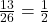## Find the probability. Enter your answer as a simplified fraction. Picking a purple marble from a jar with 13 green and 13 purple marbl

Question

Picking a purple marble from a jar with 13 green and 13 purple marbles.
The probability of picking a purple marble is

in progress 0
3 months 2021-07-24T14:52:48+00:00 1 Answers 1 views 0is your answer

Step-by-step explanation:

To calculate the probability, you first have to find the total things by adding.

13 + 13 = 26

Then, you find the number of purple marbles which is 13. So, 13 is the numerator, and 26 the denominator.Next, simplify.Have a wonderful day!